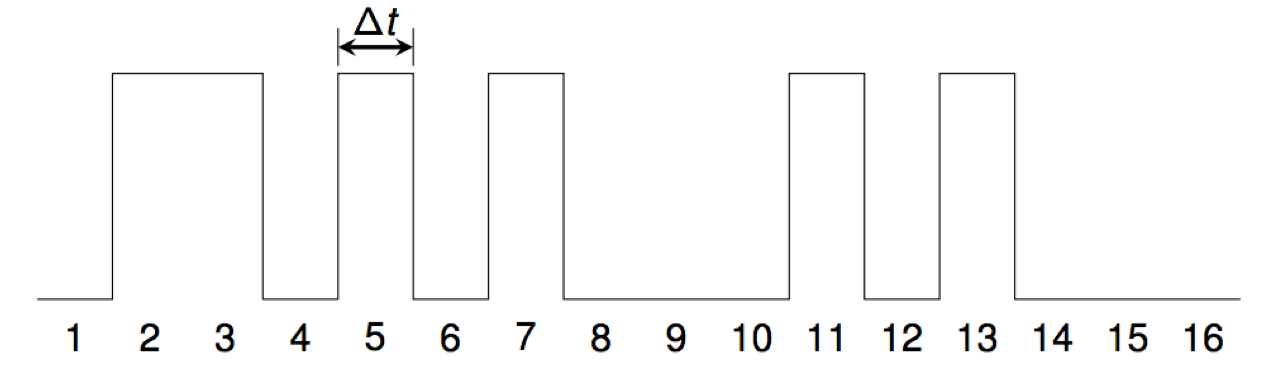# Number Theory and Noise

## Thien Do, Pubo Huang, Ally Krinsky,

This project investigates the representations of sets of positive integers (sequences) as sound.

A digital audio waveform is created from a given set A of positive integers by setting sample number i to a non-zero constant c for all i in the set. All other samples are set to zero.

For example, the waveform for the primes starts like this:We use the standard CD-audio sampling rate of 44100 samples per second, so Δt = 1/44100= 0.0000226757... seconds.

For many sets, the result is what most people would describe as noise.

## OEIS sequences

A000037 Numbers that are not squares (or, the nonsquares). download Ally Krinsky Win2020
A002328 Numbers n such that n^2-n-1 is prime. download Ally Krinsky Win2020
A002642 Numbers n such that (n^2+n+1)/3 is prime. download Ally Krinsky Win2020
A002643 Numbers n such that (n^2+n+1)/19 is prime. download Ally Krinsky Win2020
A002644 Numbers n such that (n^2+n+1)/21 is prime. download Ally Krinsky Win2020
A002731 Numbers n such that (n^2+1)/2 is prime. download Ally Krinsky Win2020
A002733 Numbers n such that (n^2 + 1)/10 is prime. download Ally Krinsky Win2020
A002970 Numbers n such that 4*n^2 + 9 is prime. download Ally Krinsky Win2020
A004083 Numbers n such that cos(n-1) <= 0 and cos(n) > 0. download Ally Krinsky Win2020
A002640 Numbers n such that (n^2 + n + 1)/3 is prime. download Ally Krinsky Win2020
A002641 Numbers n such that (n^2 + n + 1)/7 is prime. download Ally Krinsky Win2020
A002642 Numbers n such that (n^2 + n + 1)/7 is prime. download Ally Krinsky Win2020
A002643 Numbers n such that (n^2 + n + 1)/7 is prime. download Ally Krinsky Win2020
A004614 Numbers that are divisible only by primes congruent to 3 mod 4. download Ally Krinsky Win2020
A007519 Primes congruent to 1 mod 8. download Ally Krinsky Win2020 primes
A007520 Primes congruent to 3 mod 8. download Ally Krinsky Win2020 primes
A007521 Primes congruent to 5 mod 8. download Ally Krinsky Win2020 primes
A001000 a(n) = least m such that if a/b < c/d where a,b,c,d are integers in [0,n], then a/b < k/m < c/d for some integer k. download Thien Do Win2020